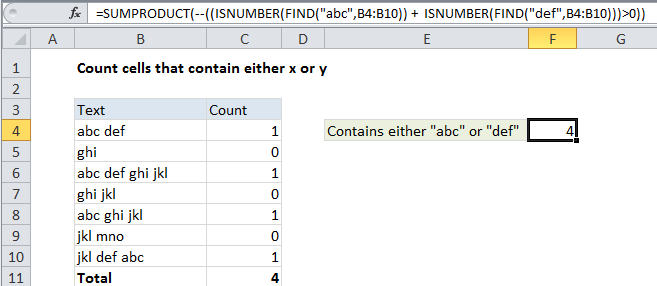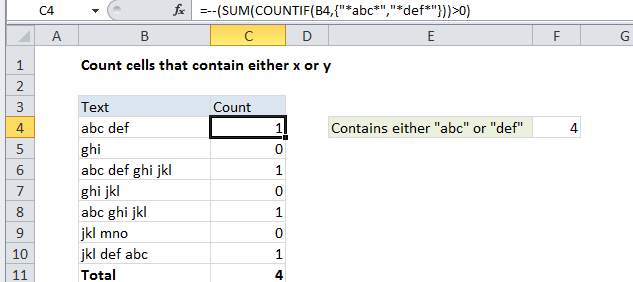## Excel Office

Excel How Tos, Tutorials, Tips & Tricks, Shortcuts

# Count cells that contain either x or y in Excel

This tutorial shows how to Count cells that contain either x or y in Excel using the example below;

## Formula

`=SUMPRODUCT(--((ISNUMBER(FIND("abc",range)) +  ISNUMBER(FIND("def",range)))>0))`## Explanation

To count cells that contain either one value or another, you an either use a helper column then tally up the count, or a more complex single cell formula.

### Background

When you count cells with “OR” criteria, you need to be careful not to double count. For example, if you are counting cells that contain “abc” or “def”, you can’t just add together two COUNTIF functions, because you may double count cells that contain both “abc” and “def”.

Worked Example:   Count cells that contain errors in Excel

### Single cell solution

For a single cell solution, you can use SUMPRODUCT with an ISNUMBER + FIND combo. The formula in cell F4 is:

`=SUMPRODUCT(--((ISNUMBER(FIND("abc",B4:B10)) +  ISNUMBER(FIND("def",B4:B10)))>0))`

This formula is based on the formula here that locates text inside of a cell:

`ISNUMBER(FIND("abc",B4:B10)`

When given a range of cells, this snippet will return an array of TRUE/FALSE values, one value for each cell the range. Since we are using this twice (once for “abc” and once for “def”), we’ll get two arrays.

Next, we add these arrays together (with +), which creates a new single array of numbers. Each number in this array is the result of adding the TRUE and FALSE values in the original two arrays together. In the example shown, the array looks like this:

Worked Example:   Count cells that do not contain in Excel

{2;0;2;0;1;0;2}

We need to add these numbers up, but we don’t want to double count. So we need to make sure any value greater than zero is just counted once. To do that, we force all values to TRUE or FALSE with “>0”, then force to 1/0 with the double-negative (–).

Finally, SUMPRODUCT adds these numbers up.

### Helper column solution

With a helper column to check each cell individually, the problem is less complex. We can use COUNTIF with two values (provided as an “array constant”). The formula in C4 is:

```=--(SUM(COUNTIF(B4,{"*abc*","*def*"}))>0)```

COUNTIF will return an array that contains two items: a count for “abc” and a count for “def”. To prevent double counting, we add the items up and then force the result to TRUE/FALSE with “>0”. Finally, we convert the TRUE/FALSE values to 1’s and 0’s with a double negative (–).

Worked Example:   Count cells that contain text in Excel

The final result is either 1 or 0 for each cell. To get a total for all cells in the range, you’ll simply sum the helper column.